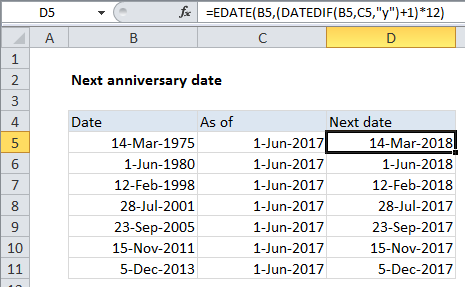## Excel Office

Excel How Tos, Tutorials, Tips & Tricks, Shortcuts

# How to calculate next anniversary date or birthday in Excel

To calculate the next anniversary date, you can use a formula based on the EDATE and DATEDIF functions.

Case Study: Assume fixing anniversary date as June 1, 2017 in all examples.

## Formula

`=EDATE(date,(DATEDIF(date,as_of,"y")+1)*12)`## Explanation

In the example shown, the formula in D5 is:

` =EDATE(B5,(DATEDIF(B5,C5,"y")+1)*12)`

This formula will work to calculate next upcoming birthday as well.

Also See:   Calculate expiration date in Excel

### How this formula works

Working from the inside out, we use the DATEDIF function to calculate how many complete years are between the the original anniversary date and the “as of” date, where the as of date is any date after the anniversary date:

`DATEDIF(B5,C5,"y")`

### As of today

To calculate the next anniversary as of today, use the TODAY() function for the as of date:

`=EDATE(date,(DATEDIF(date,TODAY(),"y")+1)*12)`

Note: Because we are interested in the *next* anniversary date, we add 1 to the DATEDIF result, then multiply by 12 to convert to years to months.

Also See:   How to get same date next year or previous year in Excel

Next, the month value goes into the EDATE function, with the original date from column B. The EDATE function rolls the original date forward by the number of months given in the previous step which creates the next upcoming anniversary date.